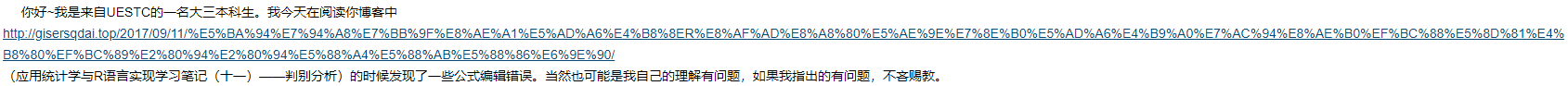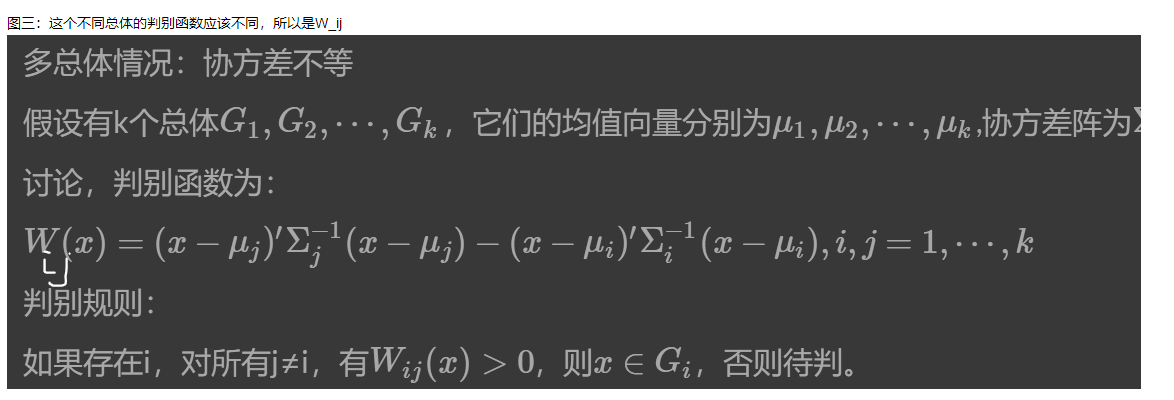0
0
01. 云栖社区>
2. 博客>
3. 正文

1 两位细心的读者2 主要问题及解决

2 来自UESTC大三小鲜肉的问题

1.这两张图是一个问题，主要是两总体协方差相等情况下的推导过程。2.这张图是关于W的下标问题。3.单位向量与投影的问题。• $\Sigma_1=\Sigma_2=\Sigma$
定义：

\begin{aligned} d^2(x,G_1)-d^2(x,G_2) & =(x-\mu_1)'\Sigma^{-1}(x-\mu_1)-(x-\mu_2)'\Sigma^{-1}(x-\mu_2) \\ &=-2[x-(\mu_1+\mu_2)/2]'\Sigma^{-1}(\mu_1-\mu_2) \end{aligned}

$x_1^{(i)},\cdots,x_{n_i}^{(i)}$为来自$G_i$的样本(i=1,2)。

$$\hat\mu^{(i)}=\frac{1}{n_i}\sum_{k=1}^{n_2}x_k^{(i)}=\bar x^{(i)},\hat \Sigma=\frac{1}{n_1+n_2-2}(S_1+S_2),$$

$$S_i=\sum_{t=1}^{n_i}(x_t^{(i)}-\bar x^{(i)})(x_t^{(i)}-\bar x^{(i)})',\bar x=\frac{1}{2}(\bar x^{(1)}+\bar x^{(2)})$$

+ 关注